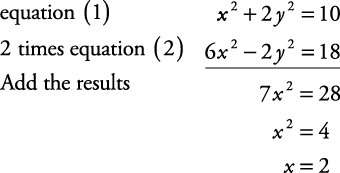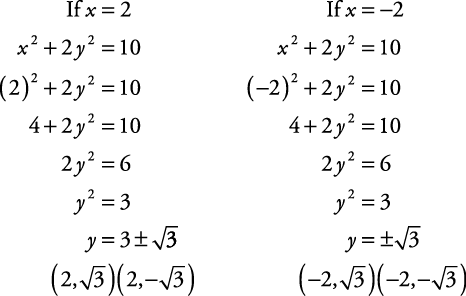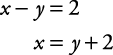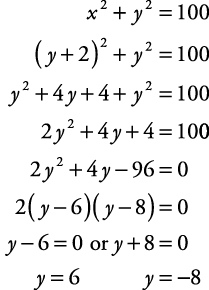## Systems of Equations Solved Algebraically

When given two equations in two variables, there are essentially two algebraic methods for solving them. One is substitution, and the other is elimination.

##### Example 1

Solve the following system of equations algebraically.

• (1)

x 2 + 2 y 2 = 10

• (2)

3 x 2y 2 = 9

This system is more easily solved using the elimination method.Using equation (1),The solution consists of the four ordered pairs. If these equations were graphed, these ordered pairs would represent the points of intersection of the graphs.

##### Example 2

Solve the following system of equations algebraically.

• (1)

x 2 + y 2 = 100

• (2)

xy = 2

This system is more easily solved using substitution. Solve equation (2) for x; then substitute that result for x in equation (1).

Solving equation (2) for x,Substituting into equation (1),Using equation (2),The solution consists of the two ordered pairs. If this system of equations were graphed, these two points would represent the points where the graphs would intersect.